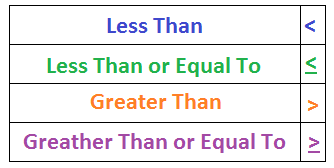# What is an Interval in Math?

An error occurred trying to load this video.

Try refreshing the page, or contact customer support.

Coming up next: Median Definition: Lesson for Kids

### You're on a roll. Keep up the good work!

Replay
Your next lesson will play in 10 seconds
• 0:58 Using Inequalities
• 1:55 Using the Number Line
• 2:31 Using Interval Notation
• 3:10 Lesson Summary
Save Save

Want to watch this again later?

Timeline
Autoplay
Autoplay
Speed Speed

#### Recommended Lessons and Courses for You

Lesson Transcript
Instructor: Allison Petrovic

Allison has experience teaching high school and college mathematics and has a master's degree in mathematics education.

In English, an interval is a period of time between events. In math, intervals are numbers that are between two other numbers. But what exactly is included and how can we use math symbols to show that? In this lesson, we'll learn what intervals are and how they can be written.

Did you hear that? The weather forecaster just said there is going to be a snowstorm with at least 3 but less than 8 inches of snow! So, what are the different amounts of snow that we could expect based on those numbers? Well, go get your snow shovel ready and then let's find out.

An interval is a range of numbers between two given numbers and includes all of the real numbers between those two numbers. As you may recall, real numbers are pretty much any number you can think of: 3.56, 171, √5, -0.157, π, etc. When the forecaster said that there would be at least 3 but less than 8 inches of snow, he described the amount of snow in an interval!

Intervals can be written using inequalities, a number line, or in interval notation! There are also special ways to indicate whether the two given numbers, known as endpoints, are included in the interval.

## Using Inequalities

The math inequalities are the symbols that stand for less than, less than or equal to, greater than, and greater than or equal to. Like these. Find the picture to recall what each of these symbols are!Let's break our weather forecast down and write the interval using inequalities:

The first part is that it will snow at least 3 inches. That means that the amount of snow, which we will represent with the variable x since it's unknown, will be at least equal to 3 but could be greater than 3. The second part of our interval is that the amount of snow, x, will be less than 8 inches.

Find the image that shows this inequality. We read this image like this: 3 is less than or equal to x, which is less than 8. So, this interval is including the endpoint 3 (because it's equal to), but not including the endpoint 8. So x is any real number from 3 all the way to the last real number before 8.

## Using the Number Line

To show an interval on a number line, you first draw two circles at the two endpoints of the interval. So, we will draw circles at 3 and 8. Now, draw a line to connect the two circles! The last step is to color in the circles only if the endpoint is included in the interval.

To unlock this lesson you must be a Study.com Member.

### Register to view this lesson

Are you a student or a teacher?

#### See for yourself why 30 million people use Study.com

##### Become a Study.com member and start learning now.
Back
What teachers are saying about Study.com

### Earning College Credit

Did you know… We have over 200 college courses that prepare you to earn credit by exam that is accepted by over 1,500 colleges and universities. You can test out of the first two years of college and save thousands off your degree. Anyone can earn credit-by-exam regardless of age or education level.